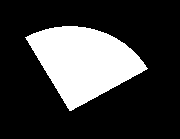# sector() function in C

• Difficulty Level : Medium
• Last Updated : 06 Dec, 2019

The header file graphics.h contains sector() function which draws and fills an elliptical pie slice with (x, y) as center, (s_angle, e_angle) as starting and ending angle and (x_radius, y_radius) as x and y radius of sector.
Syntax :

```void sector(int x, int y, int s_angle,

where,
(x, y) is center of the sector.
(s_angle, e_angle) are starting
and ending angles.
```

Examples :

```Input : x = 200, y = 200, s_angle = 0,
e_angle = 150, x_radius = 50,
Output :Input : x = 200, y = 200, s_angle = 30,
e_angle = 120, x_radius = 90,
Output :```

Below is the implementation of sector() function :

 `// C Implementation for sector()``#include `` ` `// driver code``int` `main()``{``    ``// gm is Graphics mode which is``    ``// a computer display mode that``    ``// generates image using pixels.``    ``// DETECT is a macro defined in``    ``// "graphics.h" header file``    ``int` `gd = DETECT, gm;`` ` `    ``// initgraph initializes the``    ``// graphics system by loading a``    ``// graphics driver from disk``    ``initgraph(&gd, &gm, ``""``);`` ` `    ``// sector function``    ``sector(200, 200, 0, 150, 50, 65);`` ` `    ``getch();`` ` `    ``// closegraph function closes the``    ``// graphics mode and deallocates``    ``// all memory allocated by``    ``// graphics system .``    ``closegraph();`` ` `    ``return` `0;``}`

Output :

``````
My Personal Notes arrow_drop_up